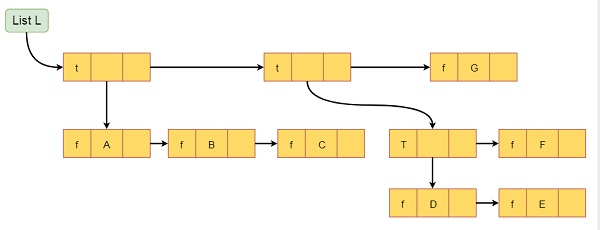# Generalized Lists in Data Structure

Data StructureAnalysis of AlgorithmsAlgorithms

In this section we will see the generalized lists. The generalized list can be defined as below −

A generalized list L is a finite sequence of n elements (n ≥ 0). The element ei is either an atom (single element) or another generalized list. The elements ei that are not atoms, they will be sub-list of L. Suppose L is ((A, B, C), ((D, E), F), G). Here L has three elements sub-list (A, B, C), sub-list ((D, E), F), and atom G. Again sub-list ((D, E), F) has two elements one sub-list (D, E) and atom F.

In C++, we can define the Generalized list structure like below −

class GeneralizedListNode{
private:
GeneralizedListNode *next;
bool tag;
union{
char data;
GeneralizedListNode *down;
};
};

So if the tag is true, then element represented by the node is a sub-list. The down points to the first node in the sub-list. If tag is false, the element is atom. The next pointer points to the next element in the list. The list will be look like this.